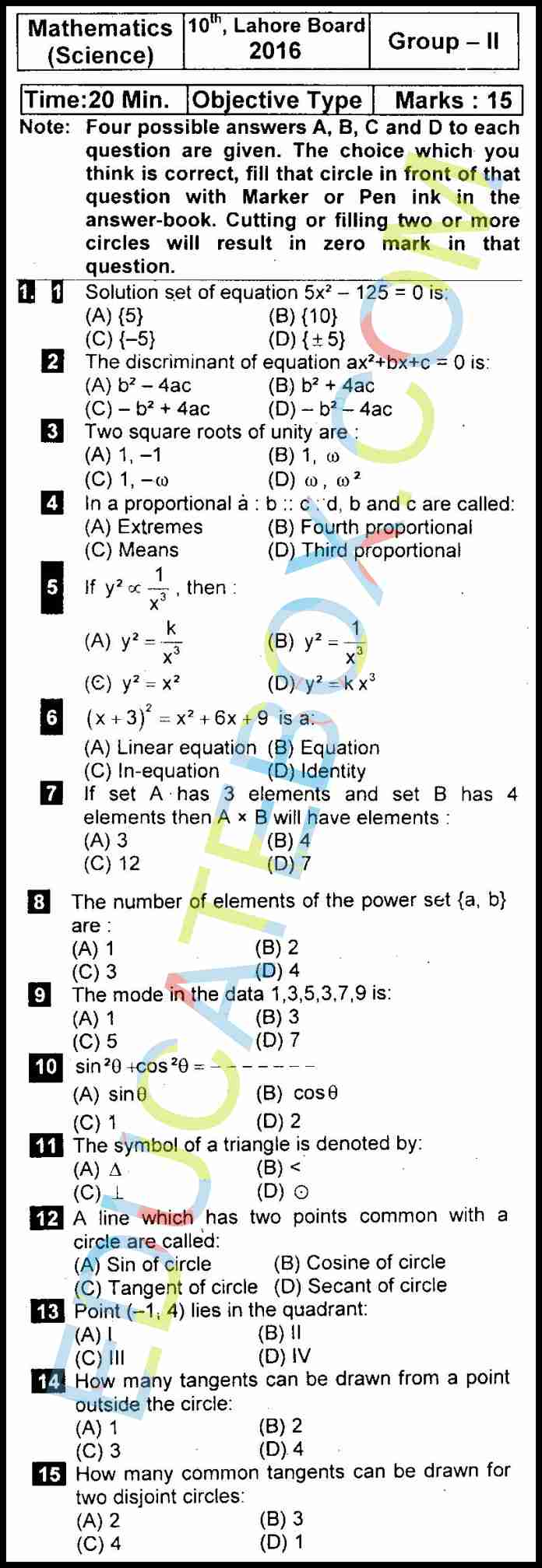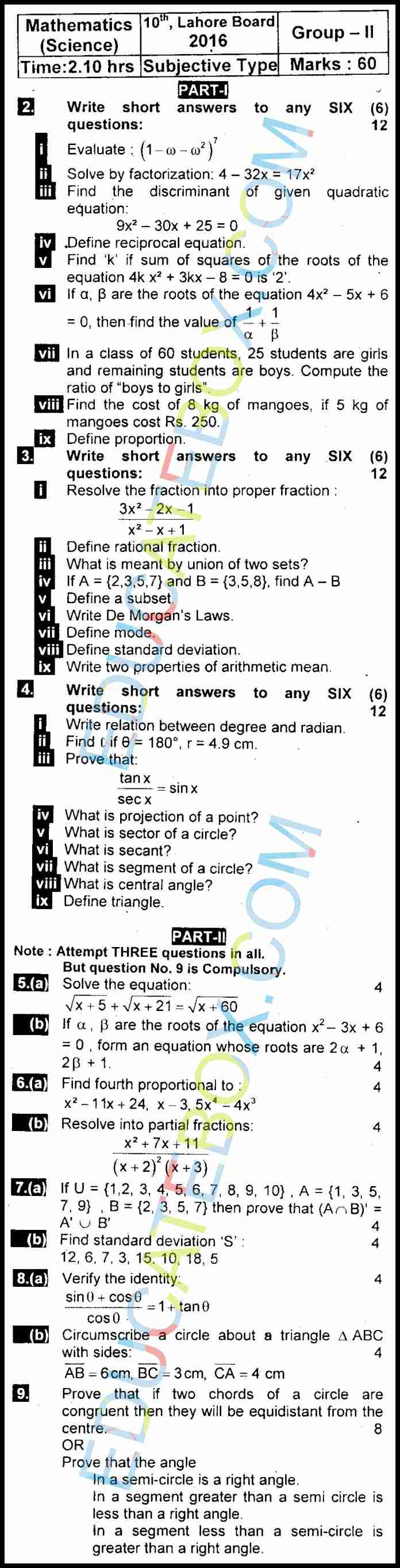Past Paper 10th Maths Lahore 2016 Group 2

0
192

Past Paper Class 10 Maths Lahore Board 2016 Objective Type Group 2 (English Medium)

Here is past paper of Maths for 10th class. It is 2016 group 2 paper, English medium version of this paper. The objective part consists of fifteen multiple choice questions. Maximum time allowed is 15 minutes. First two questions and sixth questions are solutions to the equations. There are some geometry questions and some other miscellaneous questions.Past Paper Class 10 Maths Lahore Board 2016 SUBJECTIVE Type Group 2 (English Medium)

The subjective part questions include quadratic and linear equations, ratio questions, questions of fractions, sets and laws of sets, Mode, Mean questions, and many questions from geometry portion.